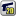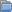﻿ Unreal Software - Thread: Pull a Player

# ForumCS2DScriptsPull a Player

#Pull a Player

14 replies
Goto Page1Mami Tomoe
User
OfflineHello!

I'm requesting assistance in the creation of a function which will do the following:Pull a player to the given X and Y coordinates.Code:
1
2
3
function pull(p, x, y, force)
-- magic(?)
endThe code should be as efficient as possible, since I'm going to call it often (at least every second).Also, a player shouldn't be pulled through a wallunless
force
is set to true.

Thanksfish
Ortimh
User
OfflineCode:
1
2
3
4
5
6
7
8
local floor = math.floor
local setpos = 'setpos %d %d %d'

function pull(p, x, y, force)
if not tile(floor(x/32), floor(y/32), 'wall') or force then
parse(setpos:format(p, x, y))
end
end
I'm awesome ... and I really like cookies.
Mami Tomoe
User
OfflineI meant that you would be able to use this in order to pull a player (p) to another player (x, y), but not instantly.

Maybe a "strength" parameter is required, though I don't mind if it's static (4 pixels are fine).
fish
Quattro
GAME BANNED
OfflineYou have to use lerp. Or if you want to make it run real fast, use bressenham's algorithm.

I'd make it using a function which moves a player a bit towards the target and runs itself with a timer which will be a way to control speed and stops when it either reaches the other player or bumps into a wall.
User
OfflineCode:
1
2
3
4
5
6
7
8
9
10
11
12
13
14
15
16
17
18
19
20
21
22
23
24
25
26
27
function move(id,start_x,start_y,amount_loop,speed,distance_x,distance_y,wallcheck)
if start_x==0 then start_x= player(id,"x") end
if start_y==0 then start_y= player(id,"y") end
timer(speed,"parse",'lua "parameter('..id..','..start_x..','..start_y..','..amount_loop..','..speed..','..distance_x..','..distance_y..','..wallcheck..')"',amount_loop)
end
function parameter(id,start_x,start_y,amount_loop,speed,distance_x,distance_y,wallcheck)
if counter==nil then counter=0 end
if counter>=amount_loop then counter=0 end
if wallcheck==nil or wallcheck<0 then wallcheck=0 end
if wallcheck>=1 then
start_x = start_x + (counter*distance_x)
start_y = start_y + (counter*distance_y)
extra_x = player(id,"tilex")
extra_y = player(id,"tiley")
if tile(extra_x,extra_y,"walkable")==true then
counter = counter + 1
parse("setpos "..id.." "..start_x.." "..start_y)
speed=speed+speed
end
elseif wallcheck==0 then
counter = counter + 1
start_x = start_x + (counter*distance_x)
start_y = start_y + (counter*distance_y)
parse("setpos "..id.." "..start_x.." "..start_y)
speed=speed+speed
end
endSkriptbasierte Bewegungen (4) has written:
Parameters:
id = Player-Id
start_x = the start position of the player in pixels (0 means the actual player position.)
start_y = the start position of the player in pixels (0 means the actual player position)
speed = in which time distance does the player move in miliseconds? (lower values means a faster and more liquid motion)
distance_x = how much pixels (x) does the player move every "speed"-miliseconds?
distance_y = how much pixels (y) does the player move every "speed"-miliseconds?
amount_loop = how often does the player moves? (higher values means that the animation needs more time)
wallcheck = set to 1 if the player should stop if he is touching a wall. Set to 0 if the player can clip through the wall.

Now we just need a giant fist grabbing the player to his destinationedited 1×, last 08.11.19 08:40:39 pm
Share time limited free games hereOrtimh
User
OfflineCode:
1
2
3
4
5
6
7
8
9
10
11
12
13
14
15
16
17
18
19
20
21
22
23
24
25
26
27
28
29
30
31
32
33
34
35
36
37
38
39
40
41
42
43
44
45
-- t = the elapsed time
-- b = begin
-- c = change (ending-beginning)
-- d = duration
local function inquad(t, b, c, d)
t = t / d
return c * (t*t) + b
end

local floor = math.floor
local parameter = 'lua "_pull%(%d,%d,%d,%d,%d,%d,%s%)'
local setpos = 'setpos %d %d %d'
local time = {} -- to determine of the elapsed time

function _pull(i, sx, sy, ex, ey, d, f)
time[i] = time[i] + 1

local x = inquad(time[i], sx, ex-sx, d)
local y = inquad(time[i], sy, ey-sy, d)

if not tile(floor(x/32), floor(y/32), 'walkable') or force then
parse(setpos:format(i, x, y))
end
end

-- id       = player ID
-- x, y     = to what position does it pull
-- duration = the duration of the pull (in seconds)
-- force    = penetrate through walls?
function pull(id, x, y, duration, force)
if not time[id] or time[id] > 0 then
time[id] = 0
end

local param = parameter:format(id,
-- i assume that player coordinates are not
-- integers
floor(player(id, 'x')),
floor(player(id, 'y')),
x, y,
duration,
force)

timer(1000, 'parse', param, duration)
end

I'm followingQuattro's suggestions andBowlinghead's code. I don't test it though, I'm sorry.
I'm awesome ... and I really like cookies.
Mami Tomoe
User
OfflineI don't think it works, is there a simple way to just pull a player towards X, Y?

Like:
pull(p, x, y, pixels, force)

Also I have a special timer function that does this:

Timer(duration, function() end, count)

So assume I'm going to use it if needed.
fish
User
OfflineI mean we gave you a full code but apparently you didnt even read through it.
Code:
1
2
3
4
5
6
-- id       = player ID
-- x, y     = to what position does it pull
-- duration = the duration of the pull (in seconds)
-- force    = penetrate through walls?
function pull(id, x, y, duration, force)
(...)

Actually to pull a player you miss a parameter which is the duration, or in other words how fast you pull the people. Or what is your definiton of force?

Whats the matter? When the code doesnt work you should at least give the red console error to us and mark the line.Ortimh has written:
I don't test it though, I'm sorry.

Me neither
edited 1×, last 09.11.19 07:46:23 pm
Share time limited free games hereMami Tomoe
User
OfflineThere's no error, the player I'm trying to pull just disappears and I don't know where he goes because I have many bots.
fish
User
OfflineYou can run 2 clients on the same windows PC for that matters.

And also you could use print, dont you.
Share time limited free games hereMami Tomoe
User
OfflineI don't think using 3 functions just makes sense, it would be much faster and easier to remake this.

What I give:

victim_id - int
goal_x - int
goal_y - int
strength_in_pixels - int
should_skip_walls - boolean
fish
User
OfflineInstructions unclear about strength in pixels;
so target will almost never hit the goal coords unless strength = sqrt(dx² + dy²)?
Share time limited free games hereMami Tomoe
User
OfflineThe target player will move x amount of pixels towards x, y.
fish
Ortimh
User
OfflineProbably the error is in the string formatting at line 22, my bad. Well what you want is clearly linear movement towards the specified coordinates, not with easing function like I did with in quad, which gives a more pull-like movement.
Code:
1
2
3
4
5
6
7
8
9
10
11
12
13
function pull(id, x, y, speed, force)
local px, py = player(id, 'x'), player(id, 'y')
local a = math.atan2(x-px, py-y) -- angle from player to x,y
local nx = px + speed * math.cos(a)
local ny = py + speed * math.sin(a)

if not tile(math.floor(nx/32), math.floor(ny/32), 'walkable') or force then
parse('setpos ' .. id .. ' ' .. nx .. ' ' .. ny)
return true
end

return false
end

It would return true if it successfully pulls the player, false if otherwise. It doesn't check whether the player have arrived to the destination or not.

You can't use a timer if you don't specify the duration of the pull, so you need to call the function as you see it fits (like every second). Well you can use a timer however you need to gain the length of player to specified coordinates and divide it with the speed, and with another kind of logic which is tiresome.
I'm awesome ... and I really like cookies.
Quattro
GAME BANNED
OfflineThis is bare bones, you can improve this by letting the player be pulled alongside wall^^
Code:
1
2
3
4
5
6
7
8
9
10
11
12
13
14
15
16
17
18
19
20
21
22
23
24
25
26
27
28
29
30
31
32
33
34
35
36
37
38
39
40
41
42
43
44
45
46
47
48
49
50
51
52
53
54
55
56
57
58
59
60
61
62
63
64
65
66
67
68
69
70
71
72
73
74
75
76
77
78
79
80
81
82
83
84
85
86
----------------------
--Setup vars----------
----------------------
function array(size, value)
local array = {}
for i = 1, size do
array[i] = value
end
return array
end
player_pull = array(32, false)

----------------------
--Functions-----------
----------------------
function wordSplit(input, separator)
if separator == nil then
separator = "%s"
end
local t = {} ; i = 1
for str in string.gmatch(input, "([^"..separator.."]+)") do
t[i] = str
i = i + 1
end
return t
end
function pull(infostring) --infostring: {id targetlocationX targetlocationY speed force} lower speed = faster

local sensitivity = 2 --pixels to move each check. increasing this adds performance and makes the pull choppy.
local wall_distance = 16 --pixels to stop before getting sucked into a wall

--do not change these
local var = wordSplit(infostring)
local id = tonumber(var)
local x = tonumber(var)
local y = tonumber(var)
local speed = tonumber(var)
local force = var
local px, py = player(id, 'x'), player(id, 'y')
local vectorX = x - px
local vectorY = y - py
local magnitude = math.sqrt(vectorX^2 + vectorY^2)
local dirX = vectorX / magnitude
local dirY = vectorY / magnitude
local targetX = px + dirX * sensitivity
local targetY = py + dirY * sensitivity
local checkX = px + dirX * wall_distance
local checkY = py + dirY * wall_distance

if magnitude < wall_distance or not player_pull[id] then
return
end

if force == '1' then
parse('setpos ' .. id .. ' ' .. targetX .. ' ' .. targetY)
timer(speed, 'pull', id..' '..x..' '..y..' '..speed..' '..force, 1)
else
if tile(math.floor(checkX / 32), math.floor(checkY / 32), 'walkable') then
parse('setpos ' .. id .. ' ' .. targetX .. ' ' .. targetY)
timer(speed, 'pull', id..' '..x..' '..y..' '..speed..' '..force, 1)
return
else
timer(speed, 'pull', id..' '..x..' '..y..' '..speed..' '..force, 1)
return
end
end
end

----------------------
--Hook Functions------
----------------------
function serveraction(id, action)
if action == 1 then
if not player_pull[id] then
player_pull[id] = true
pull(id..' 0 0 25 0')
else
player_pull[id] = false
end
end
end

----------------------
--Hooks---------------
----------------------1